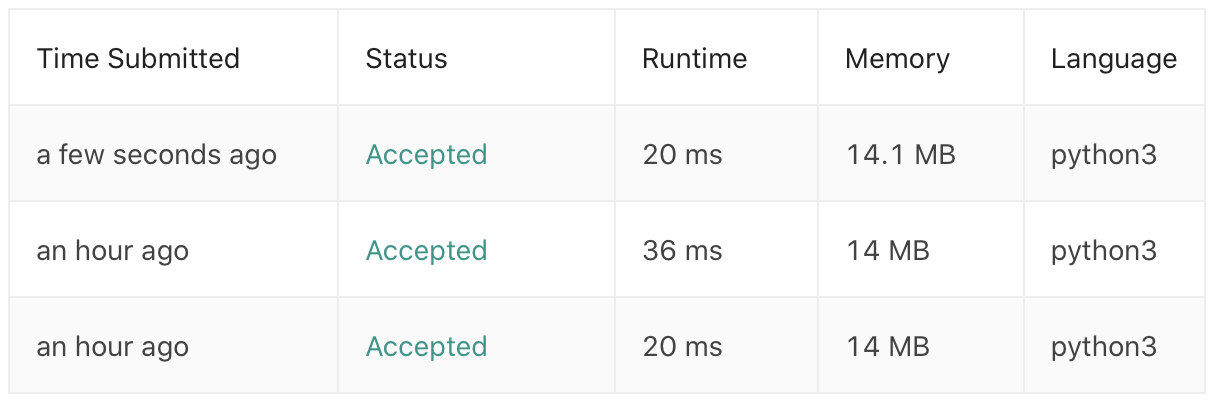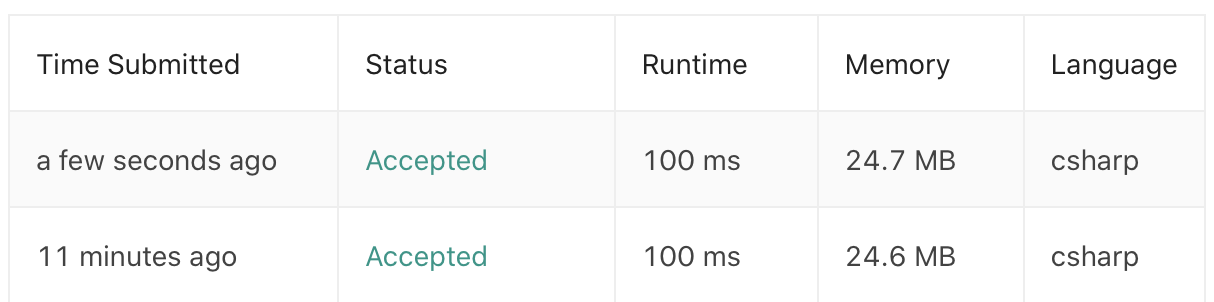LeetCode | 5. Longest Common Prefix 最长公共前缀PuranLongest Common Prefix 是 LeetCode 算法题库中的第十四道题，难度为 Easy，题目地址为：https://leetcode.com/problems/longest-common-prefix/

﻿

1. 问题描述

﻿

Input: [“flower”,”flow”,”flight”]
Output: “fl”

2. 解题思路

﻿

1. 纵向扫描：从前往后遍历所有字符串的每一列，比较相同列上的字符是否相同，如果相同则继续对下一列进行比较，如果不同则当前列不再属于公共前缀，当前列之前的部分为最长公共前缀。

2. 横向扫描：依次遍历每个字符串，对于每个字符串，更新最长公共前缀，当遍历完所有字符串以后，就可以得到最长公共前缀了。该方法是基于这样的基础：

3. 分治：类似于横向扫描方法，将字符串数组分为拆成两个子部分来计算最长公共前缀。

4. 二分查找：通过找到字符串数组中的最短数组，以这个字符串的长度为基础取得中间值mid，判断每个字符串的长度为mid 的前缀是否相同。

• 分治

• 二分查找

﻿

4. 代码

﻿

Python 实现

• 纵向扫描

class Solution:
def longestCommonPrefix(self, strs: List[str]) -> str:
if not strs:
return ""
length, count = len(strs), len(strs)
for i in range(length):
c = strs[i]
for j in range(1, count):
if (i == len(strs[j]) or strs[j][i] != c):
return strs[:i]
return strs

﻿

• 横向扫描

class Solution:
def longestCommonPrefix(self, strs: List[str]) -> str:
if not strs:
return ""
prefix, count = strs, len(strs)
for i in range(1, count):
prefix = self.lcp(prefix, strs[i])
if not prefix:
break;
return prefix
def lcp(self, str1, str2):
length, index = min(len(str1), len(str2)), 0
while index < length and str1[index] == str2[index]:
index += 1
return str1[:index]

﻿

• 分治

class Solution:
def longestCommonPrefix(self, strs: List[str]) -> str:
def lcp(start, end):
if start == end:
return strs[start]
mid = (start + end) // 2
lcpLeft, lcpRight = lcp(start, mid), lcp(mid + 1, end)
minLength = min(len(lcpLeft), len(lcpRight))
for i in range(minLength):
if (lcpLeft[i] != lcpRight[i]):
return lcpLeft[:i]
return lcpLeft[:minLength]
return "" if not strs else lcp(0, len(strs) - 1)

﻿﻿

C# 实现

• ‍纵向扫描

public class Solution {
public string LongestCommonPrefix(string[] strs) {
if (strs == null || strs.Length == 0)
return "";
int length = strs.Length;
int count = strs.Length;
string prefix = strs;
for (int i = 0; i < length; i++)
{
char c = strs[i];
for (int j = 0; j < count; j++)
{
if (i == strs[j].Length || strs[j][i] != c)
{
prefix = prefix.Substring(0, i);
return prefix;
}
}
}
return prefix;
}
}

﻿

• ‍横向扫描

public class Solution {
public string LongestCommonPrefix(string[] strs) {
if (strs == null || strs.Length == 0)
return "";
int count = strs.Length;
string prefix = strs;
for (int i = 1; i < count; i++)
{
prefix = lcp(prefix, strs[i]);
}
return prefix;
}
string lcp(string str1, string str2)
{
int length = Math.Min(str1.Length, str2.Length);
int index = 0;
while (index < length && str1[index] == str2[index])
{
++index;
}
return str1.Substring(0, index);
}
}

﻿﻿Puran

GIS从业者，正在往开发的路上小跑。 2018.03.29 加入

评论